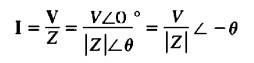Hi, friends welcome to new post. In this post, we discuss Real, Reactive, And Apparent Power In Ac Circuits. In ac electrical power system there is three types of power used that are reactive real and apparent power. All these powers have their own effect at the system and define their operation

In this post, we discuss all parameters related to these powers with the details. So let get started.

## Real, Reactive, And Apparent Power In Ac Circuits

• In dc circuitry is shown in the below figure.• The power given to the dc load is multiple of voltage about load and current passing in it.

p = VI

• In the case of ac circuits having a sine waveform is complicated since there is an angle difference among the ac volts and ac current given to the load.
• The instantaneous power given to the ac load is the multiple of instantaneous voltage and instances current. With that average power given to the load effect through phase angle among the voltage and current.
• Here we discuss the factor of phase difference at the average power given to the ac load.
• The shown below indicates the one phase voltage power source giving power to the single-phase load having impedance Z=Z∠θ ohm.
• If we suppose that the load is inductor it caused the angle of θ of the load to be positive and current is lagging the voltage through zero degrees.
• The voltage given to load is.

v(t) = √V cos wt

• Here V denotes the rms value of voltage given to the load and resultant current passing has value.

i(t) = ∨2I COS(wt-θ)

• Here I is the rms value of current passing in the load.
• The instantaneous power given to the load for given time t is mention here,

p(t) = v(t)i(t) = 2VI cos wt COS(wt – (θ)

• The angle θ in the above equation is the impedance angle of the laod.
• In case of inductive load the impedance angle has a positive value and current signal lagging the voltage through angle θ.
• After application of trigonometric to the above equation then we have.

p(t) = VI cos (θ) (1 + cos 2wt) + VI sin (θ) sin 2wt—-B

p = Vl cosθ

• That is real power given to the load through the equation shown B. The unit that denotes real power is watts and denoted as W.
• We can see that 2nd terminology of the instantaneous power equation is positive half of time and negative portion of time.due to this casue the net power is 0.
• This terminology is denoted power which is ist moved from power source to the load after that goes through laod to the power supply source.
• The power components that is moving among the load and power supply source is called reactive power and denotes with Q.
• The reactive power denotes power that is ist saved and after that uses in the magnetic field of the inductor.
• The equation of reactive power is

Q=VIsinθ

• In this equation, θ denotes the impedance angle of load. Q is positive in case of inductive loads and negative in case of capacitive loads since the impedance angle is positive for inductive loads and has negative values for capacitive loads
• The units of reactive powers are volt-ampere. dimensional units has a similar value for watts and reactive power.
• The apparent power S is given to the load is explained the multiple voltages about the load and current in the load
• It is the power that looks to be given the laod if phase angle difference between the voltage and current is diminished.
• So the apparent power of load is mentioned here.
• S=VI

### Other Types of Power Equations

• If the impedance is constant value the ohm law will be used to run other equations for real apparent and reactive powers given to the load.
• As the magnitude of voltage about the load is mentioned here.
• V=IZ
• now using this equation in the above expression we have.### What is Complex Power

• For easy understanding, real reactive powers denote in complex power denoted as S.
• s =P + jQ
• The equation of complex power is given here.
• S = VI*

### Relationships between Impedance Angle, Current Angle, and Power

• According to simple circuitry configuration, the inductive load offer a positive value of impedance angle• Reactance of the inductor is positive if the impedance angle of the load has a positive value  the phase angle of current passing in the load is lagging the phase angle of voltage about the load through θ### What is Power Angle

• The real reactive and apparent power is given to the load is correspondent to the power triangle.
• The power triangle can be seen here.• The angle in the more left-down portion is the impedance angle. The neighboring part of the triangle is real power given to the load the opposite part of the triangle is reactive power Q given to the load and hypotenuse of this triangle shows the apparent power S of the load.
• The term cos θ is generally called power factor. The P.F is explained as a fraction of apparent power S which is given to the real power load.
• PF = cos θ
• Here θ denotes the impedance angle

That is a detailed post about Real, Reactive, And Apparent Power In Ac Circuits if you have any query ask in the comments. See you in next post.